Prealgebra

# 4.6Add and Subtract Mixed Numbers

Prealgebra4.6 Add and Subtract Mixed Numbers

### Learning Objectives

By the end of this section, you will be able to:
• Model addition of mixed numbers with a common denominator
• Add mixed numbers with a common denominator
• Model subtraction of mixed numbers
• Subtract mixed numbers with a common denominator
• Add and subtract mixed numbers with different denominators

### Be Prepared 4.6

Before you get started, take this readiness quiz.

1. Draw figure to model $73.73.$
If you missed this problem, review Example 4.6.
2. Change $114114$ to a mixed number.
If you missed this problem, review Example 4.9.
3. Change $312312$ to an improper fraction.
If you missed this problem, review Example 4.11.

### Model Addition of Mixed Numbers with a Common Denominator

So far, we’ve added and subtracted proper and improper fractions, but not mixed numbers. Let’s begin by thinking about addition of mixed numbers using money.

If Ron has $11$ dollar and $11$ quarter, he has $114114$ dollars.

If Don has $22$ dollars and $11$ quarter, he has $214214$ dollars.

What if Ron and Don put their money together? They would have $33$ dollars and $22$ quarters. They add the dollars and add the quarters. This makes $324324$ dollars. Because two quarters is half a dollar, they would have $33$ and a half dollars, or $312312$ dollars.

$114+214________324=312114+214________324=312$

$114+214114+214$

We can use fraction circles to model this same example:

 $114+214114+214$ Start with $114114$. one whole and one $1414$ pieces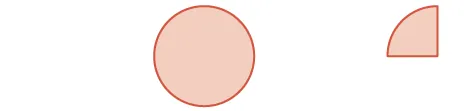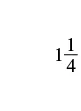Add $214214$ more. two wholes and one $1414$ pieces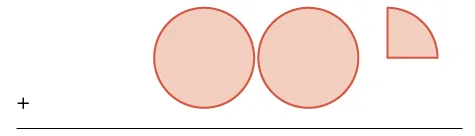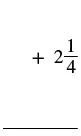The sum is: three wholes and two $1414$'s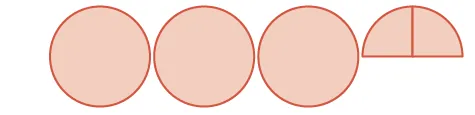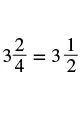### Example 4.81

Model $213+123213+123$ and give the sum.

### Try It 4.161

Use a model to add the following. Draw a picture to illustrate your model.

$125+335125+335$

### Try It 4.162

Use a model to add the following. Draw a picture to illustrate your model.

$216+256216+256$

### Example 4.82

Model $135+235135+235$ and give the sum as a mixed number.

### Try It 4.163

Model, and give the sum as a mixed number. Draw a picture to illustrate your model.

$256+156256+156$

### Try It 4.164

Model, and give the sum as a mixed number. Draw a picture to illustrate your model.

$158+178158+178$

Modeling with fraction circles helps illustrate the process for adding mixed numbers: We add the whole numbers and add the fractions, and then we simplify the result, if possible.

### How To

#### Add mixed numbers with a common denominator.

Step 1. Add the whole numbers.

Step 3. Simplify, if possible.

### Example 4.83

Add: $349+229.349+229.$

### Try It 4.165

Find the sum: $447+127.447+127.$

### Try It 4.166

Find the sum: $2311+5611.2311+5611.$

In Example 4.83, the sum of the fractions was a proper fraction. Now we will work through an example where the sum is an improper fraction.

### Example 4.84

Find the sum: $959+579.959+579.$

### Try It 4.167

Find the sum: $878+758.878+758.$

### Try It 4.168

Find the sum: $679+859.679+859.$

An alternate method for adding mixed numbers is to convert the mixed numbers to improper fractions and then add the improper fractions. This method is usually written horizontally.

### Example 4.85

Add by converting the mixed numbers to improper fractions: $378+438.378+438.$

### Try It 4.169

Find the sum by converting the mixed numbers to improper fractions:

$559+379.559+379.$

### Try It 4.170

Find the sum by converting the mixed numbers to improper fractions:

$3710+2910.3710+2910.$

Table 4.2 compares the two methods of addition, using the expression $325+645325+645$ as an example. Which way do you prefer?

Mixed Numbers Improper Fractions
$325+6459659+659+1151015325+6459659+659+1151015$ $325+645175+3455151015325+645175+3455151015$
Table 4.2

### Model Subtraction of Mixed Numbers

Let’s think of pizzas again to model subtraction of mixed numbers with a common denominator. Suppose you just baked a whole pizza and want to give your brother half of the pizza. What do you have to do to the pizza to give him half? You have to cut it into at least two pieces. Then you can give him half.

We will use fraction circles (pizzas!) to help us visualize the process.Algebraically, you would write: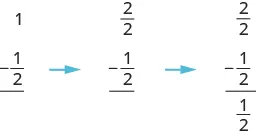### Example 4.86

Use a model to subtract: $1−13.1−13.$

### Try It 4.171

Use a model to subtract: $1−14.1−14.$

### Try It 4.172

Use a model to subtract: $1−15.1−15.$

What if we start with more than one whole? Let’s find out.

### Example 4.87

Use a model to subtract: $2−34.2−34.$

### Try It 4.173

Use a model to subtract: $2−15.2−15.$

### Try It 4.174

Use a model to subtract: $2−13.2−13.$

In the next example, we’ll subtract more than one whole.

### Example 4.88

Use a model to subtract: $2−125.2−125.$

### Try It 4.175

Use a model to subtract: $2−113.2−113.$

### Try It 4.176

Use a model to subtract: $2−114.2−114.$

What if you start with a mixed number and need to subtract a fraction? Think about this situation: You need to put three quarters in a parking meter, but you have only a $11$ bill and one quarter. What could you do? You could change the dollar bill into $44$ quarters. The value of $44$ quarters is the same as one dollar bill, but the $44$ quarters are more useful for the parking meter. Now, instead of having a $11$ bill and one quarter, you have $55$ quarters and can put $33$ quarters in the meter.

This models what happens when we subtract a fraction from a mixed number. We subtracted three quarters from one dollar and one quarter.

We can also model this using fraction circles, much like we did for addition of mixed numbers.

### Example 4.89

Use a model to subtract: $114−34114−34$

### Try It 4.177

Use a model to subtract. Draw a picture to illustrate your model.

$113−23113−23$

### Try It 4.178

Use a model to subtract. Draw a picture to illustrate your model.

$115−45115−45$

### Subtract Mixed Numbers with a Common Denominator

Now we will subtract mixed numbers without using a model. But it may help to picture the model in your mind as you read the steps.

### How To

Subtract mixed numbers with common denominators.
1. Step 1. Rewrite the problem in vertical form.
2. Step 2.
Compare the two fractions.
• If the top fraction is larger than the bottom fraction, go to Step 3.
• If not, in the top mixed number, take one whole and add it to the fraction part, making a mixed number with an improper fraction.
3. Step 3. Subtract the fractions.
4. Step 4. Subtract the whole numbers.
5. Step 5. Simplify, if possible.

### Example 4.90

Find the difference: $535−245.535−245.$

### Try It 4.179

Find the difference: $649−379.649−379.$

### Try It 4.180

Find the difference: $447−267.447−267.$

Just as we did with addition, we could subtract mixed numbers by converting them first to improper fractions. We should write the answer in the form it was given, so if we are given mixed numbers to subtract we will write the answer as a mixed number.

### How To

#### Subtract mixed numbers with common denominators as improper fractions.

Step 1. Rewrite the mixed numbers as improper fractions.

Step 2. Subtract the numerators.

Step 3. Write the answer as a mixed number, simplifying the fraction part, if possible.

### Example 4.91

Find the difference by converting to improper fractions:

$9611−71011.9611−71011.$

### Try It 4.181

Find the difference by converting the mixed numbers to improper fractions:

$649−379.649−379.$

### Try It 4.182

Find the difference by converting the mixed numbers to improper fractions:

$447−267.447−267.$

### Add and Subtract Mixed Numbers with Different Denominators

To add or subtract mixed numbers with different denominators, we first convert the fractions to equivalent fractions with the LCD. Then we can follow all the steps we used above for adding or subtracting fractions with like denominators.

### Example 4.92

Add: $212+523.212+523.$

### Try It 4.183

Add: $156+434.156+434.$

### Try It 4.184

Add: $345+812.345+812.$

### Example 4.93

Subtract: $434−278.434−278.$

### Try It 4.185

Find the difference: $812−345.812−345.$

### Try It 4.186

Find the difference: $434−156.434−156.$

### Example 4.94

Subtract: $3511−434.3511−434.$

### Try It 4.187

Subtract: $134−678.134−678.$

### Try It 4.188

Subtract: $1037−2249.1037−2249.$

### Section 4.6 Exercises

#### Practice Makes Perfect

In the following exercises, use a model to find the sum. Draw a picture to illustrate your model.

436.

$1 1 5 + 3 1 5 1 1 5 + 3 1 5$

437.

$2 1 3 + 1 1 3 2 1 3 + 1 1 3$

438.

$1 3 8 + 1 7 8 1 3 8 + 1 7 8$

439.

$1 5 6 + 1 5 6 1 5 6 + 1 5 6$

Add Mixed Numbers with a Common Denominator

440.

$5 1 3 + 6 1 3 5 1 3 + 6 1 3$

441.

$2 4 9 + 5 1 9 2 4 9 + 5 1 9$

442.

$4 5 8 + 9 3 8 4 5 8 + 9 3 8$

443.

$7 9 10 + 3 1 10 7 9 10 + 3 1 10$

444.

$3 4 5 + 6 4 5 3 4 5 + 6 4 5$

445.

$9 2 3 + 1 2 3 9 2 3 + 1 2 3$

446.

$6 9 10 + 8 3 10 6 9 10 + 8 3 10$

447.

$8 4 9 + 2 8 9 8 4 9 + 2 8 9$

Model Subtraction of Mixed Numbers

In the following exercises, use a model to find the difference. Draw a picture to illustrate your model.

448.

$1 1 6 − 5 6 1 1 6 − 5 6$

449.

$1 1 8 − 5 8 1 1 8 − 5 8$

Subtract Mixed Numbers with a Common Denominator

In the following exercises, find the difference.

450.

$2 7 8 − 1 3 8 2 7 8 − 1 3 8$

451.

$2 7 12 − 1 5 12 2 7 12 − 1 5 12$

452.

$8 17 20 − 4 9 20 8 17 20 − 4 9 20$

453.

$19 13 15 − 13 7 15 19 13 15 − 13 7 15$

454.

$8 3 7 − 4 4 7 8 3 7 − 4 4 7$

455.

$5 2 9 − 3 4 9 5 2 9 − 3 4 9$

456.

$2 5 8 − 1 7 8 2 5 8 − 1 7 8$

457.

$2 5 12 − 1 7 12 2 5 12 − 1 7 12$

Add and Subtract Mixed Numbers with Different Denominators

In the following exercises, write the sum or difference as a mixed number in simplified form.

458.

$3 1 4 + 6 1 3 3 1 4 + 6 1 3$

459.

$2 1 6 + 5 3 4 2 1 6 + 5 3 4$

460.

$1 5 8 + 4 1 2 1 5 8 + 4 1 2$

461.

$7 2 3 + 8 1 2 7 2 3 + 8 1 2$

462.

$9 7 10 − 2 1 3 9 7 10 − 2 1 3$

463.

$6 4 5 − 1 1 4 6 4 5 − 1 1 4$

464.

$2 2 3 − 3 1 2 2 2 3 − 3 1 2$

465.

$2 7 8 − 4 1 3 2 7 8 − 4 1 3$

Mixed Practice

In the following exercises, perform the indicated operation and write the result as a mixed number in simplified form.

466.

$2 5 8 · 1 3 4 2 5 8 · 1 3 4$

467.

$1 2 3 · 4 1 6 1 2 3 · 4 1 6$

468.

$2 7 + 4 7 2 7 + 4 7$

469.

$2 9 + 5 9 2 9 + 5 9$

470.

$1 5 12 ÷ 1 12 1 5 12 ÷ 1 12$

471.

$2 3 10 ÷ 1 10 2 3 10 ÷ 1 10$

472.

$13 5 12 − 9 7 12 13 5 12 − 9 7 12$

473.

$15 5 8 − 6 7 8 15 5 8 − 6 7 8$

474.

$5 9 − 4 9 5 9 − 4 9$

475.

$11 15 − 7 15 11 15 − 7 15$

476.

$4 − 3 4 4 − 3 4$

477.

$6 − 2 5 6 − 2 5$

478.

$9 20 ÷ 3 4 9 20 ÷ 3 4$

479.

$7 24 ÷ 14 3 7 24 ÷ 14 3$

480.

$9 6 11 + 7 10 11 9 6 11 + 7 10 11$

481.

$8 5 13 + 4 9 13 8 5 13 + 4 9 13$

482.

$3 2 5 + 5 3 4 3 2 5 + 5 3 4$

483.

$2 5 6 + 4 1 5 2 5 6 + 4 1 5$

484.

$8 15 · 10 19 8 15 · 10 19$

485.

$5 12 · 8 9 5 12 · 8 9$

486.

$6 7 8 − 2 1 3 6 7 8 − 2 1 3$

487.

$6 5 9 − 4 2 5 6 5 9 − 4 2 5$

488.

$5 2 9 − 4 4 5 5 2 9 − 4 4 5$

489.

$4 3 8 − 3 2 3 4 3 8 − 3 2 3$

#### Everyday Math

490.

Sewing Renata is sewing matching shirts for her husband and son. According to the patterns she will use, she needs $238238$ yards of fabric for her husband’s shirt and $118118$ yards of fabric for her son’s shirt. How much fabric does she need to make both shirts?

491.

Sewing Pauline has $314314$ yards of fabric to make a jacket. The jacket uses $223223$ yards. How much fabric will she have left after making the jacket?

492.

Printing Nishant is printing invitations on his computer. The paper is $812812$ inches wide, and he sets the print area to have a $112112$-inch border on each side. How wide is the print area on the sheet of paper?

493.

Framing a picture Tessa bought a picture frame for her son’s graduation picture. The picture is $88$ inches wide. The picture frame is $258258$ inches wide on each side. How wide will the framed picture be?

#### Writing Exercises

494.

Draw a diagram and use it to explain how to add $158+278.158+278.$

495.

Edgar will have to pay $3.753.75$ in tolls to drive to the city.

Explain how he can make change from a $1010$ bill before he leaves so that he has the exact amount he needs.

How is Edgar’s situation similar to how you subtract $10−334?10−334?$

496.

Add $4512+3784512+378$ twice, first by leaving them as mixed numbers and then by rewriting as improper fractions. Which method do you prefer, and why?

497.

Subtract $378−4512378−4512$ twice, first by leaving them as mixed numbers and then by rewriting as improper fractions. Which method do you prefer, and why?

#### Self Check

After completing the exercises, use this checklist to evaluate your mastery of the objectives of this section.

After reviewing this checklist, what will you do to become confident for all objectives?

Order a print copy

As an Amazon Associate we earn from qualifying purchases.